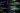# Javascript TDD with ChatGPT

April 5th, 2023
Test-driven development in Javascript with ChatGPT-4 works. An example demonstrates it using a precise description and refined prompt engineering.Previously, I wrote about programming with ChatGPT in an iterative approach followed by TDD with Python. Now that ChatGPT-4 is available, it is time to revisit the TDD (Test Driven Development) approach with Javascript for a change.

The setup is the same. Write the tests for an ASIN check function and then implement it. However, I now use ChatGPT-4, not 3.5 and some refined prompts.

## ChatGPT TDD prompting

The strategy is to define the behaviour and constraints, not the tests, first. They are language-independent, and we can generate any language from this in the following steps.

`> The function is_asin takes a string and returns a boolean. It returns true if the passed parameter adheres to the definition of an ASIN (Amazon Standard Identification Number). An ASIN is a ten-character long alphanumeric identifier with chars being uppercase. `

• `Create a list of unit tests for a function called is_asin`

• `Start the list with a happy path example`

• `Do not assume a programming language`

• `Do not show any code`

• `Use best practices`

• `Be concise, i.e. use as few tests as sensible`

Most items are self-explanatory. A simple yet important point is asking to use best practices. It commonly increases the code quality, resulting in professional styles, structures and code instead of simple beginner or tutorial solutions. The answer is a list of test cases described in plain English.

Next, we ask for the unit tests.

`> Implement the unit tests in Javascript.`

The resulting tests are exhaustive and yet simple tying down the behaviour nicely.

`> Here's a sample implementation of the unit tests using JavaScript and the Jest testing framework:`

`const is_asin = require('./is_asin'); // Assuming the function is exported from is_asin.js`

`describe('is_asin', () => {  test('Happy path test', () => {    expect(is_asin('B07H2M8ZJ6')).toBe(true);  });`

`  test('Length test - shorter than 10 characters', () => {    expect(is_asin('B07H2M8')).toBe(false);  });`

`  test('Length test - longer than 10 characters', () => {    expect(is_asin('B07H2M8ZJ65')).toBe(false);  });`

`  test('Case sensitivity test', () => {    expect(is_asin('b07H2M8ZJ6')).toBe(false);  });`

`  test('Non-alphanumeric characters test', () => {    expect(is_asin('B07H2M8*J6')).toBe(false);  });`

`  test('Empty string test', () => {    expect(is_asin('')).toBe(false);  });`

`  test('Null input test', () => {    expect(is_asin(null)).toBe(false);  });`

`  test('Unicode characters test', () => {    expect(is_asin('B07H2MöZJ6')).toBe(false);  });`

`  test('Numeric-only test', () => {    expect(is_asin('1234567890')).toBe(true);  });`

`  test('Alpha-only test', () => {    expect(is_asin('ABCDEFGHIJ')).toBe(true);  });});`

`Remember to have Jest installed in your project by running npm install --save-dev jest, and include the is_asin function implementation in a separate file called is_asin.js.`

Next, we ask for the code with a reminder to do its best and document it.

`> Implement is_asin using best practices including documentation.`

ChatGPT provides a decent, documented, working solution. Professional software engineers will be able to improve this further. In fact, ChatGPT can, too, if prompted.

`> Here's an implementation of the is_asin function using best practices and including documentation:`

`/** * Checks if the given string is a valid Amazon Standard Identification Number (ASIN). * * @param {string} input - The string to check for ASIN validity. * @returns {boolean} True if the input string is a valid ASIN, false otherwise. */function is_asin(input) {  // Check if input is a string  if (typeof input !== 'string') {    return false;  }`

`  // Check if input has the correct length  if (input.length !== 10) {    return false;  }`

`  // Check if input contains only uppercase alphanumeric characters  const uppercaseAlphanumericRegex = /^[A-Z0-9]*\$/;  return uppercaseAlphanumericRegex.test(input);}`

`module.exports = is_asin;`

`This implementation includes a function description, parameter description, and return value description in the form of JSDoc comments. The function also checks for edge cases, such as the input not being a string, having an incorrect length, or not containing only uppercase alphanumeric characters.`

## Conclusion

It is impressive how ChatGPT is progressing. At the same time, it strongly depends on the user correctly prompting, which currently takes some experience and skill. Compared to the previous example, this Javascript TDD ChatGPT-4 solution worked on the first attempt. But it did use a newer version, and I used improved prompts too.

Christian Prokopp, BoldData.org Founder

#### Let's talk

You have a business problem in need for data and analysis? Send us an email.